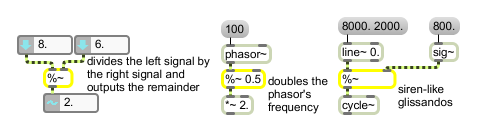# %~

Divide two signals, output the remainder

## Description

%~ is a signal remainder operator. If signals are connected to both inlets, the left signal is divided by the right signal, and the remainder is output. If a signal is only connected to left inlet, it is divided to the argument or a float in the right inlet. Note that multiple signals in the same inlet add together automatically.

## Arguments

Name Type Opt Description
initial-divisor float or int opt Sets an initial value by which to divide the signal coming into the left inlet. If a signal is connected to the right inlet, the argument is ignored. If no argument is present, and no signal is connected to the right inlet, the initial value is 1 by default.

## Messages

 int dividend/divisor [int] In left inlet: The number is divided by the signal coming into the right inlet. If a signal is also connected to the left inlet, a float or int is ignored. In right inlet: A number by which to divide the signal coming into the left inlet. If a signal is also connected to the right inlet, a float or int is ignored. float dividend/divisor [float] In left inlet: The number is divided by the signal coming into the right inlet. If a signal is also connected to the left inlet, a float or int is ignored. In right inlet: A number by which to divide the signal coming into the left inlet. If a signal is also connected to the right inlet, a float or int is ignored. signal In left inlet: The signal is divided by a signal coming into the right inlet, or a constant value received in the right inlet, and the remainder is sent out the outlet. In right inlet: The signal is used as the divisor, to be divided into the signal coming into the left inlet, or the constant value received in the left inlet.

## Output

signal: When the two signals in the inlets are divided, the remainder is sent out the outlet. % is called the modulo operator.

## Examples